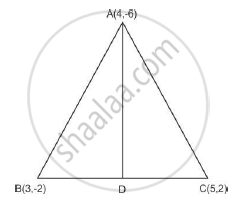# A(4, - 6), B(3,- 2) and C(5, 2) are the vertices of a 8 ABC and AD is its median. Prove that the median AD divides Δ ABC into two triangles of equal areas. - Mathematics

A(4, - 6), B(3,- 2) and C(5, 2) are the vertices of a 8 ABC and AD is its median. Prove that the median AD divides Δ ABC into two triangles of equal areas.

#### Solution

Solution:
Let the co-ordinates of D be D (x,y) and D is midpoint of BC
x = (3+5)/2 = 4; y = (2-2)/2 = 0Now Area of triangle ABD = 1/2 [4(-2-0) +3(0+6)+4(-6+2)] = 3

and Area of triangle ACD =1/2 [5(-6-0) + 4(0 - 2) + 4(2+6)] = 3

Hence, the median AD divides triangle ABC into two triangle of equal area.

Concept: Area of a Triangle
Is there an error in this question or solution?
2013-2014 (March) All India Set 2

Share